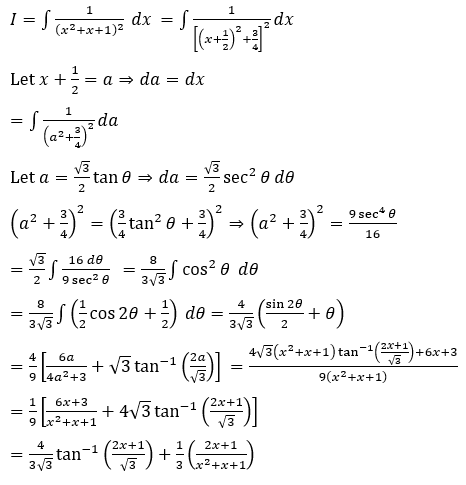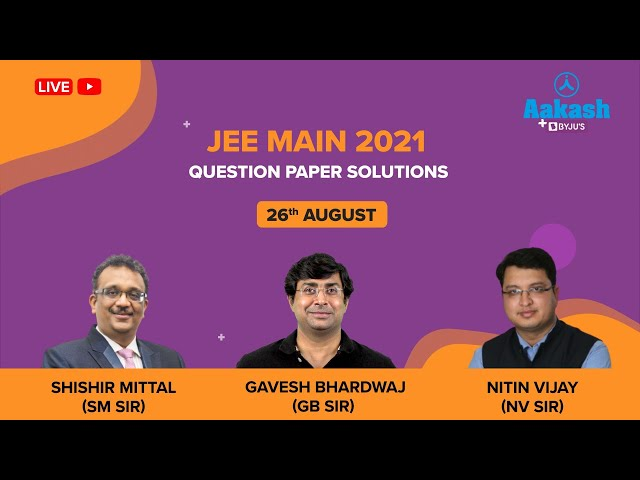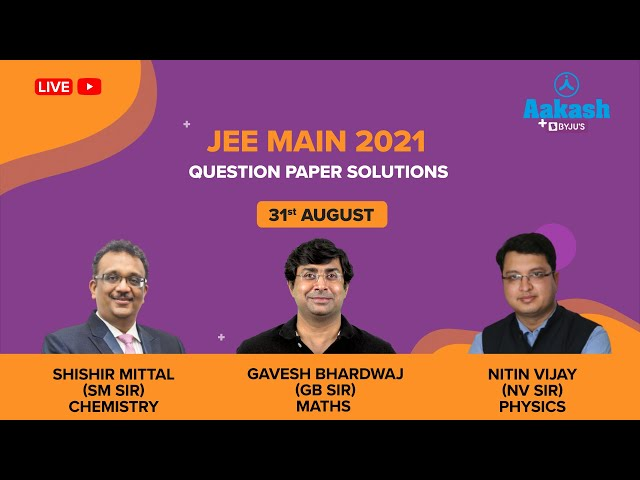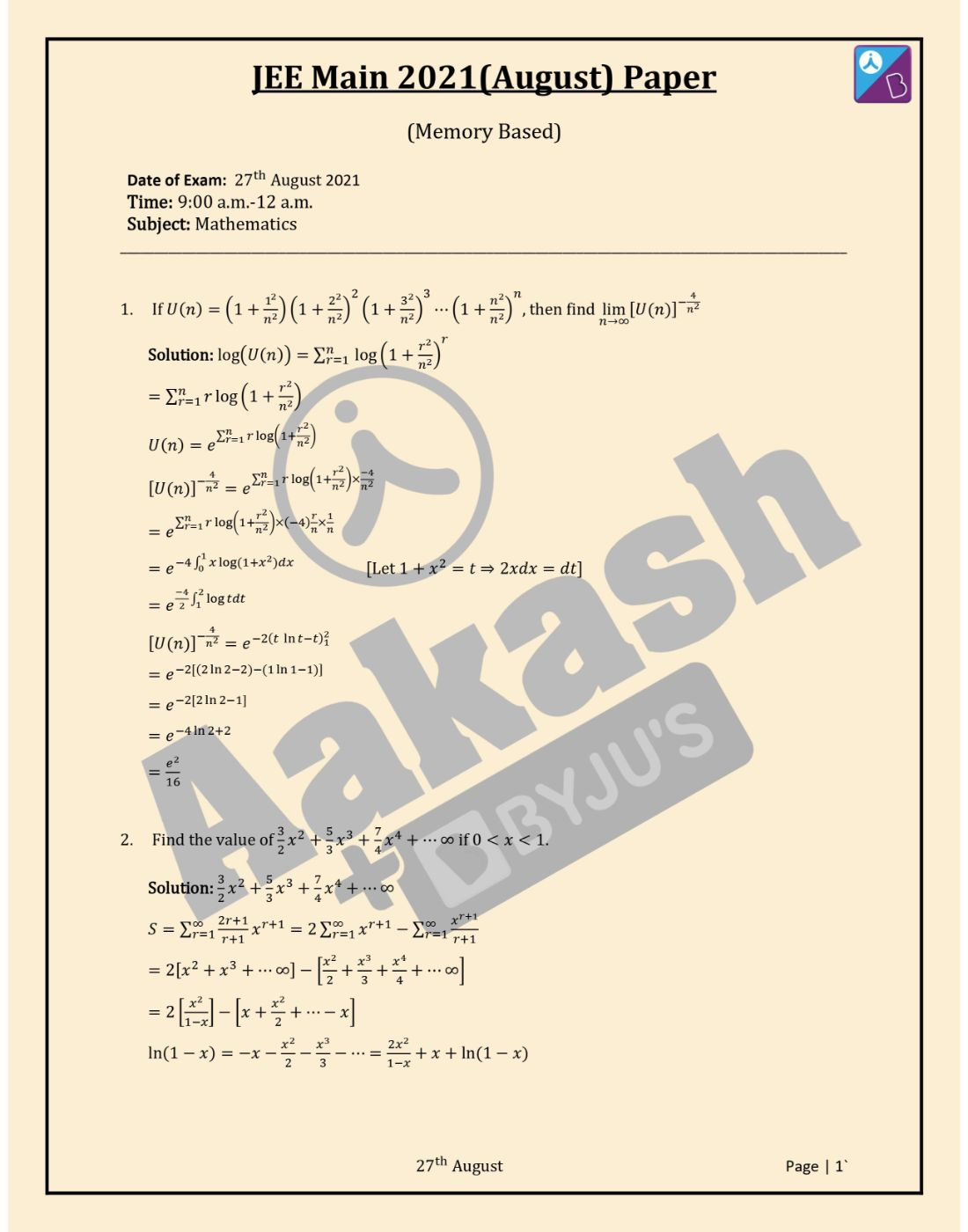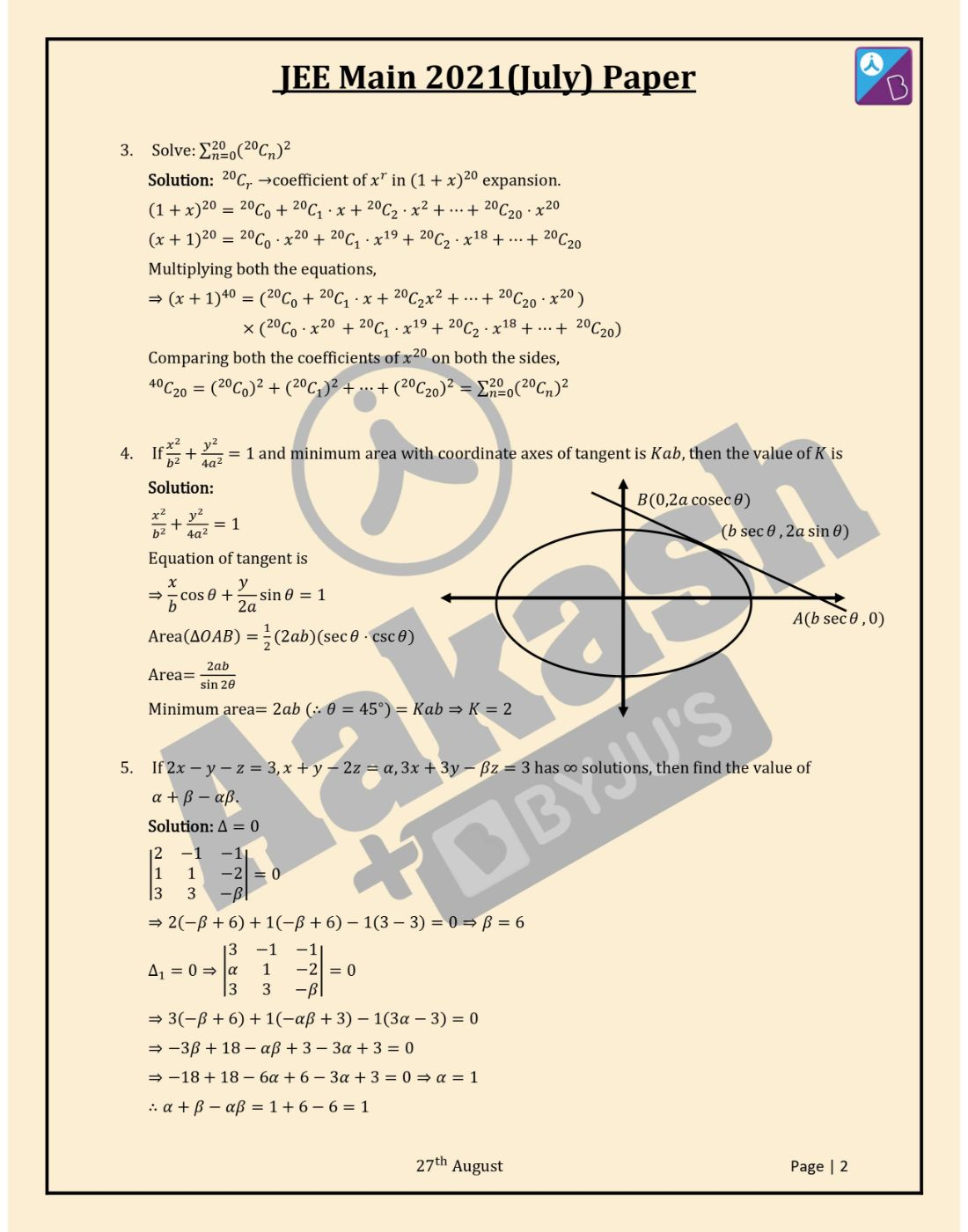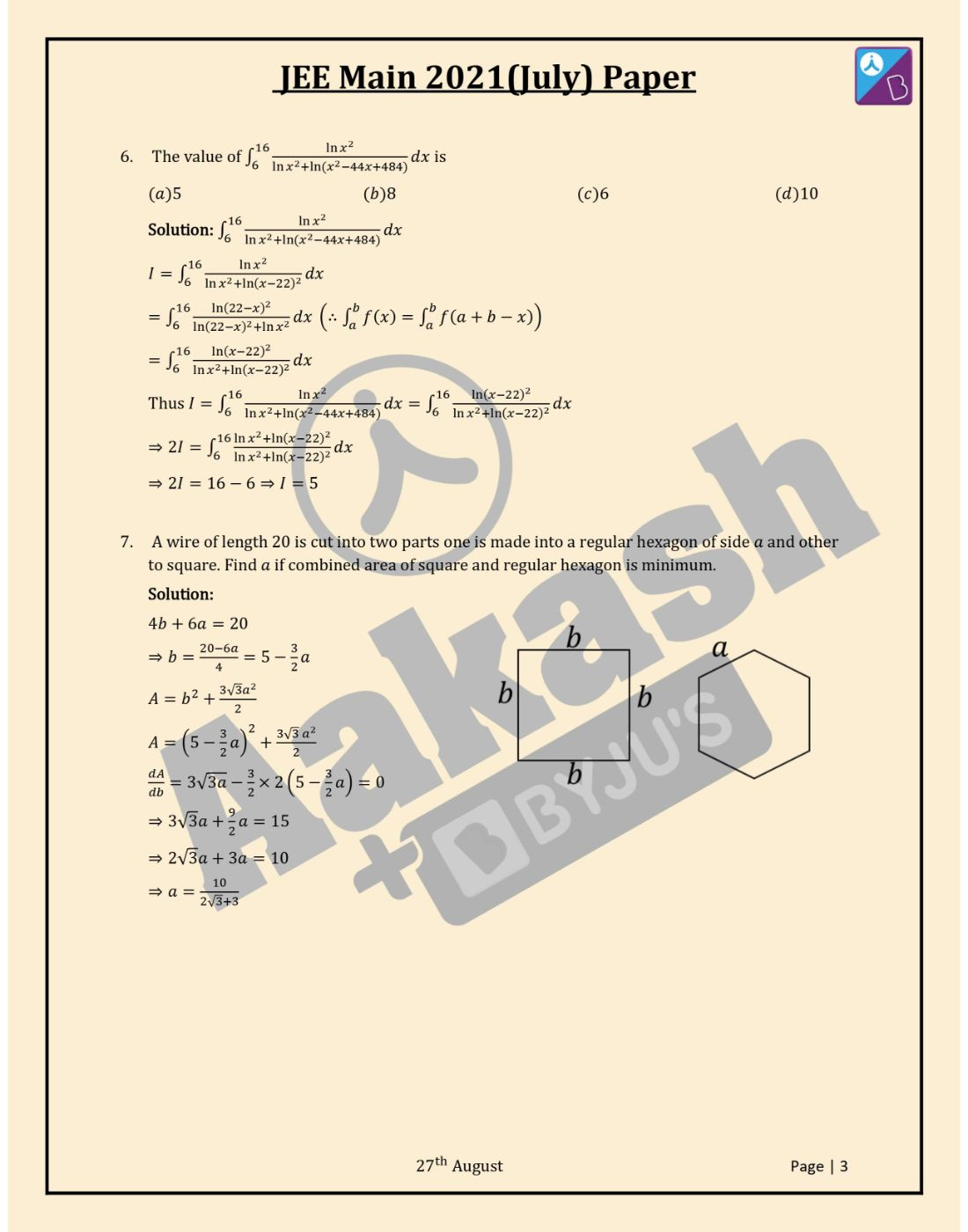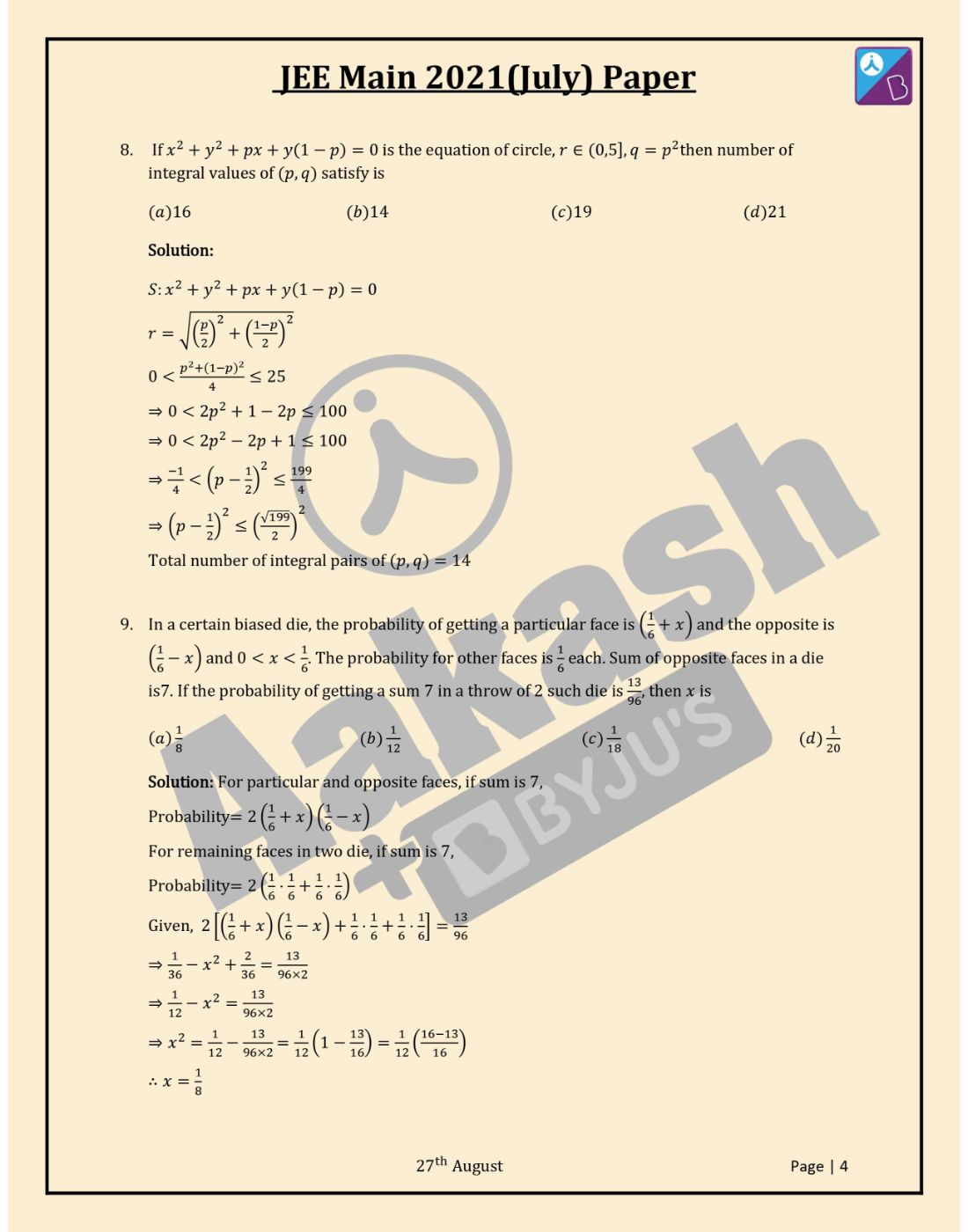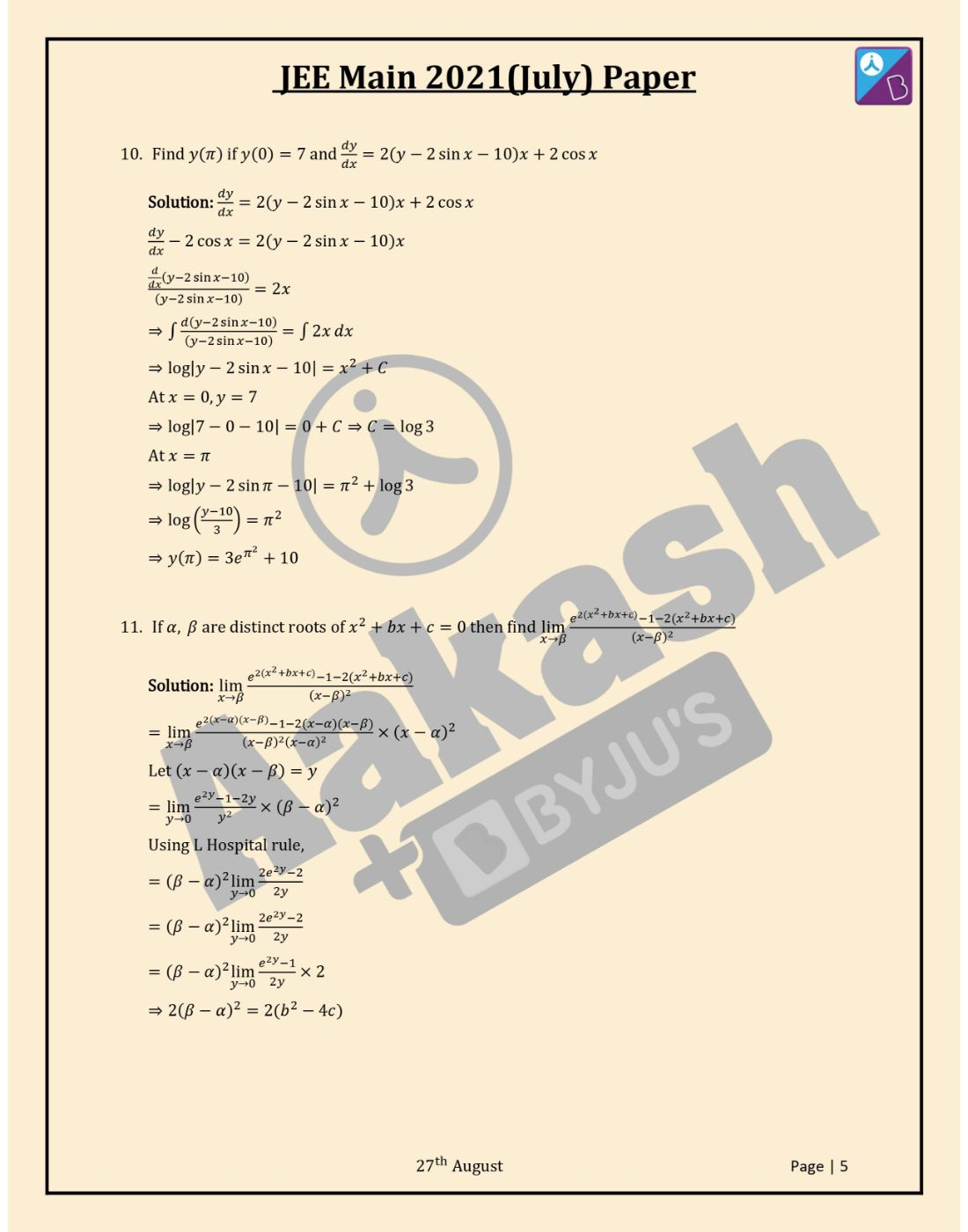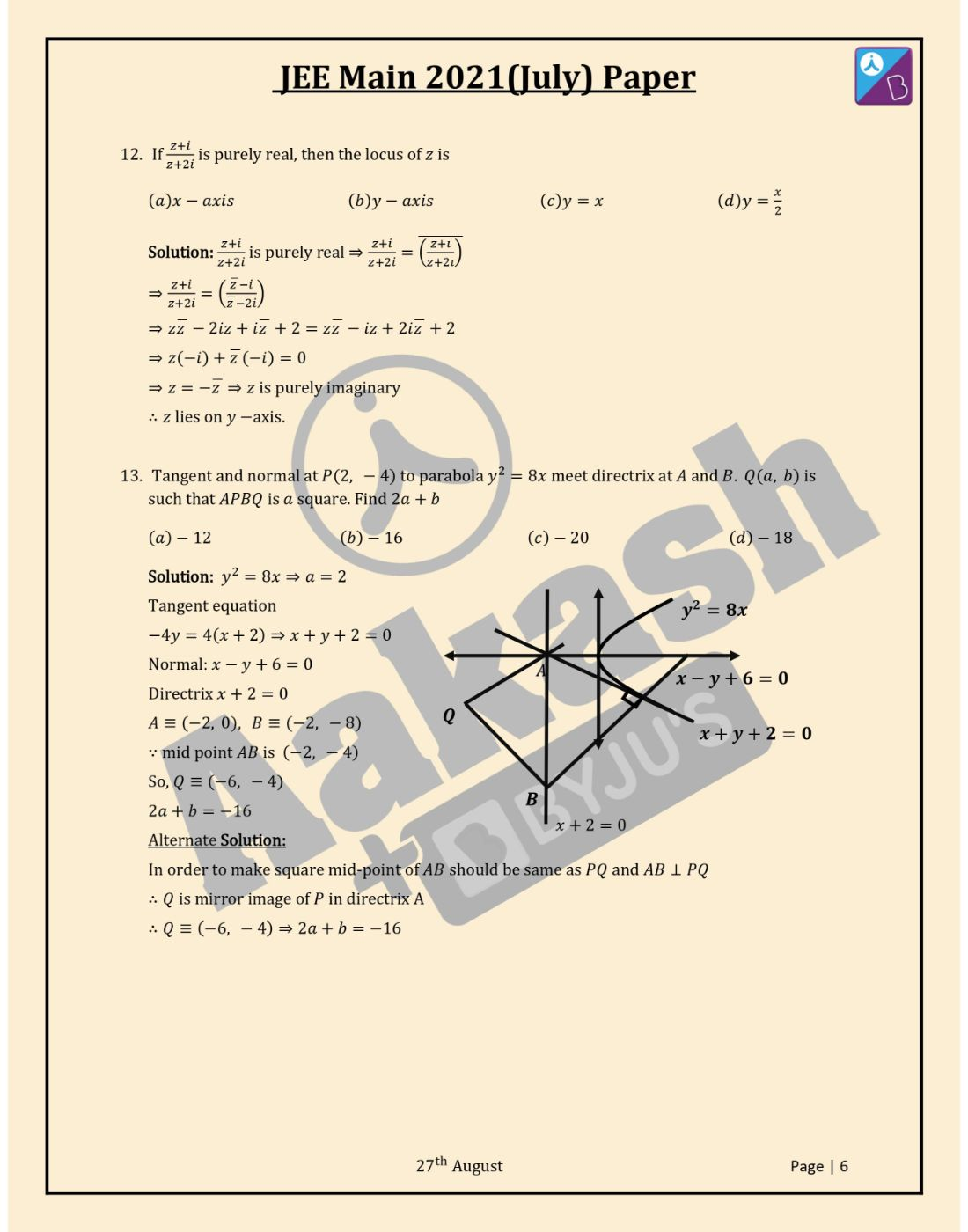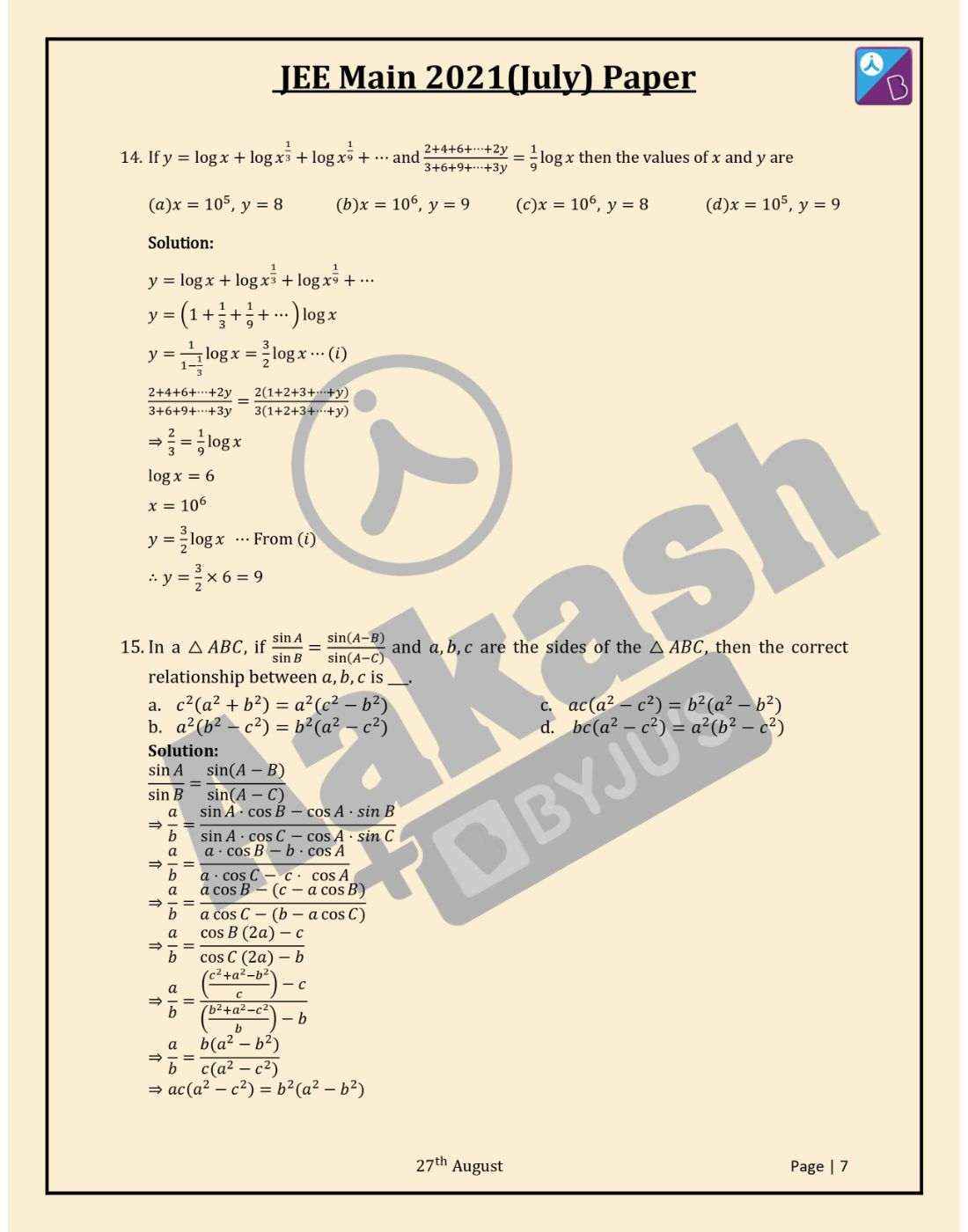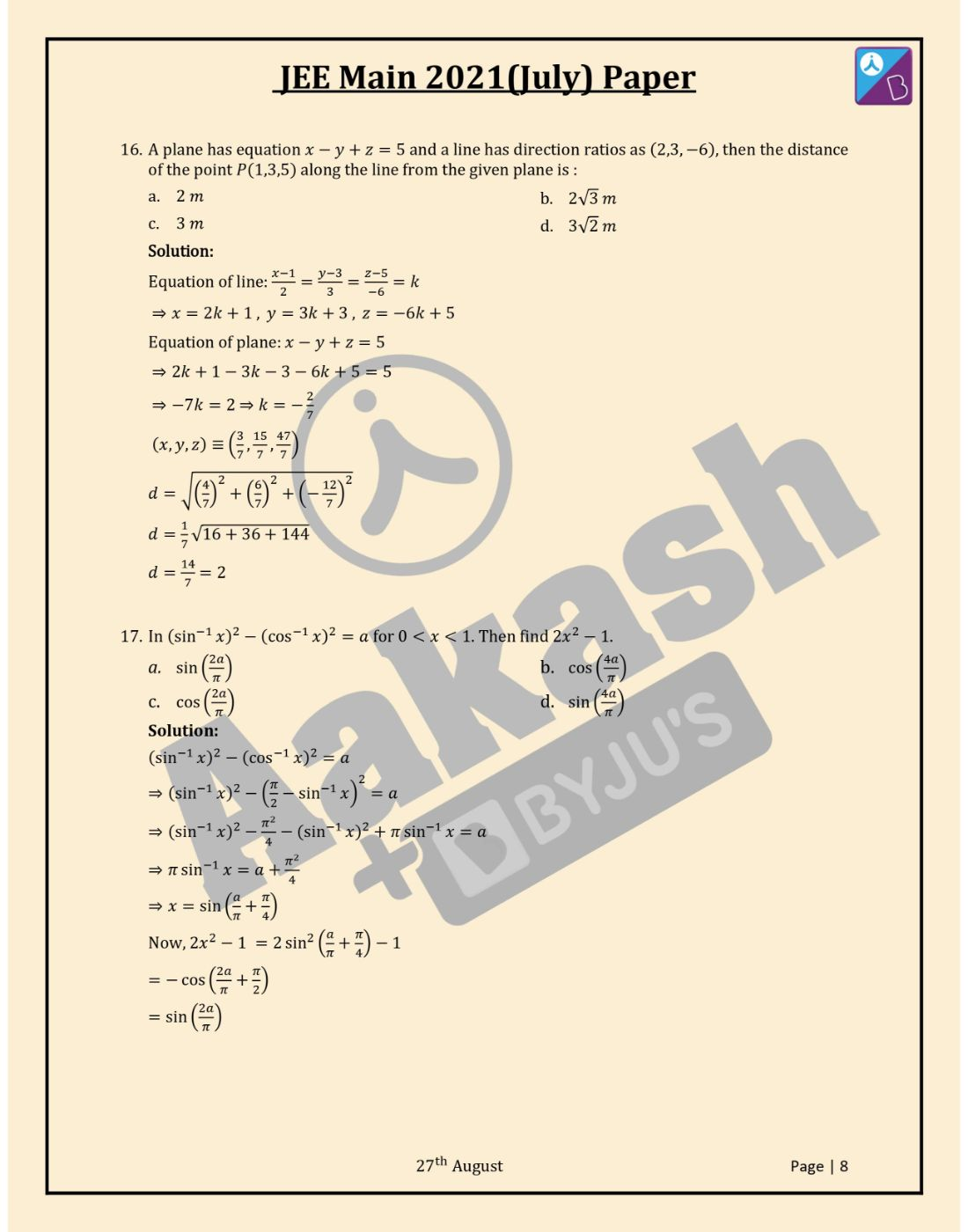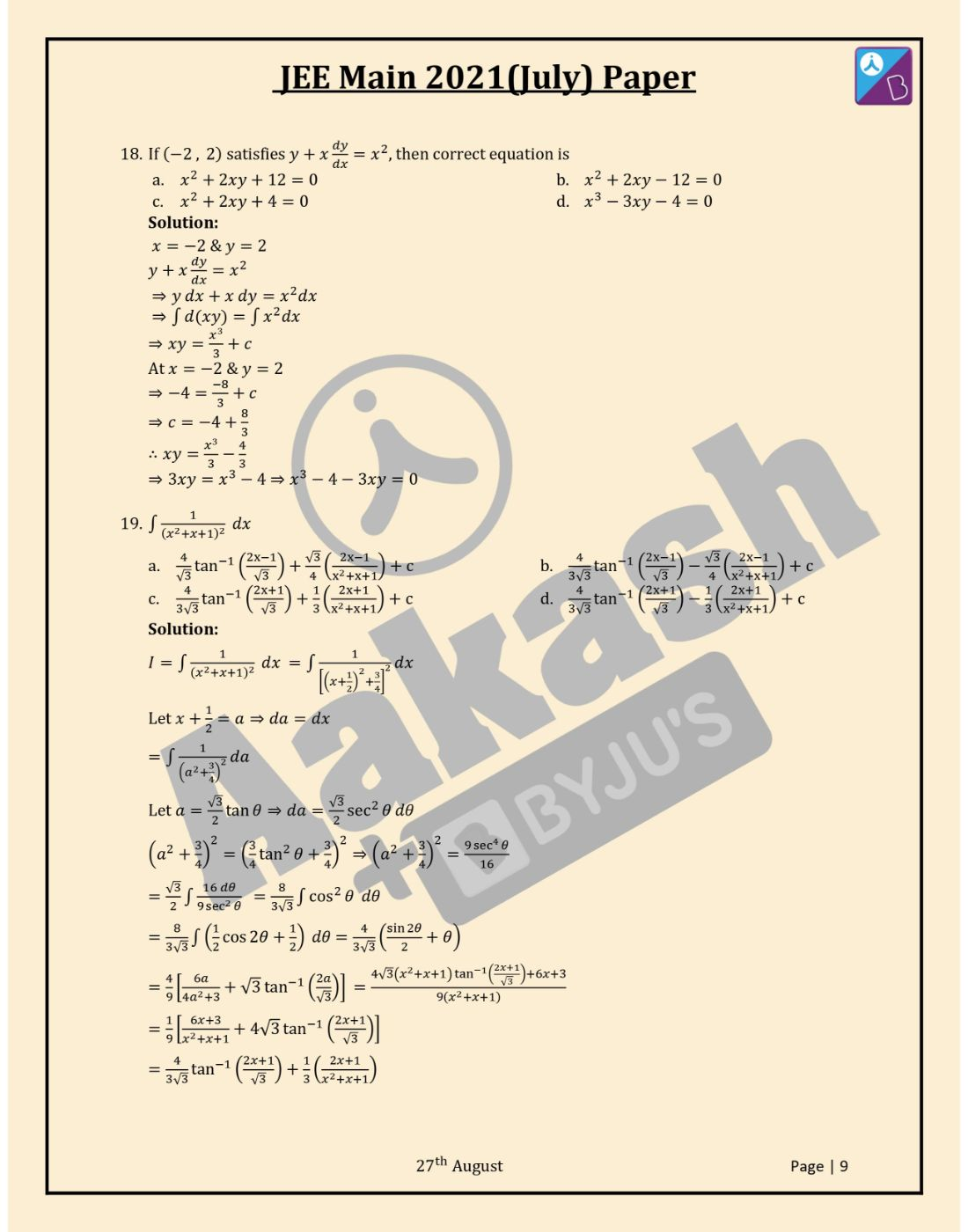Win up to 100% scholarship on Aakash BYJU'S JEE/NEET courses with ABNAT Win up to 100% scholarship on Aakash BYJU'S JEE/NEET courses with ABNAT

# JEE Main 2021 August 27 Shift 1 Maths Question Paper with Solutions

JEE Main 2021 Maths question paper for August 27 shift 1 is available here for free downloading in pdf format. JEE Main candidates must focus on solving and answering these questions to apprehend the level of the exam. Solving JEE Main 2021 Maths question papers help students to understand the exam pattern, weightage of questions and difficulty level of the exam. These solutions are designed by expert teachers at BYJU’S. Official question papers of JEE Main 2021 session 4 (August 27) will be available on the NTA website jeemain.nta.nic.in. Keep learning and stay tuned for further updates on the JEE Main Exam. Download BYJU’S App and subscribe to YouTube channel to access interactive Maths, Physics and Chemistry Videos.
JEE Main 2021 August 27th Shift 1 Maths Question Paper

Question 1:If

$$\begin{array}{l}U(n) =(1+\frac{1^2}{n^2})(1+\frac{2^2}{n^2})^2(1+\frac{3^2}{n^2})^3…..(1+\frac{n^2}{n^2})^n\end{array}$$
, then find
$$\begin{array}{l}\lim_{n \rightarrow \infty}[U(n)]^{\frac{-4}{n^2}}\end{array}$$

$$\begin{array}{l}log(U(n)) = \sum_{r=1}^n log(1+\frac{r^2}{n^2})^r\end{array}$$

=

$$\begin{array}{l}\sum_{r=1}^n r log(1+\frac{r^2}{n^2})\end{array}$$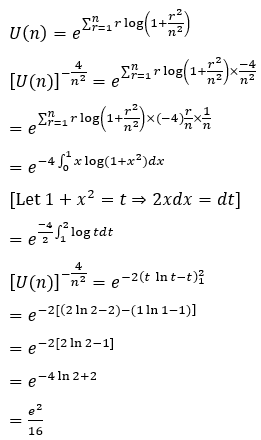Question 2: Find the value of

$$\begin{array}{l}\frac{3}{2} x^2 + \frac{5}{3} x^3 + \frac{7}{4} x^4 +……\infty\end{array}$$
if 0 < x < 1.

Let S =

$$\begin{array}{l}\sum_{r=1}^{\infty}\frac{2r+1}{r+1}x^{r+1}=2 \sum_{r=1}^{\infty}x^{r+1} – \sum_{r=1}^{\infty}\frac{x^{r+1}}{r+1}\end{array}$$

= 2[x2 + x3 + ….∞] – [x2/2 + x3/3 + x4/4 + …∞]

= 2[x2/(1-x)] – [x + x2/2 + …. – x]

ln(1-x) = -x – x2/2 – x3/3 – x4/4 – …∞] = 2x2/(1-x) + x + ln(1-x)

Question 3: Solve

$$\begin{array}{l}\sum_{n=0}^{20} (^{20}C_n)^2\end{array}$$

$$\begin{array}{l}\sum_{n=0}^{20} (^{20}C_n)^2\end{array}$$
$$\begin{array}{l}(^{20}C_r) \rightarrow\end{array}$$
coefficient of xr in (1+x)20 expansion.

$$\begin{array}{l}(1+x)^{20}=^{20}C_0+^{20}C_1x+^{20}C_2x^2+ ..+^{20}C_{20}x^{20}\end{array}$$
$$\begin{array}{l}(x+1)^{20}=^{20}C_0 x^{20} + ^{20}C_1x^{19} +^{20}C_2x^{18}+ ..+^{20}C_{20}\end{array}$$

Multiplying both the equations,

$$\begin{array}{l}(x+1)^{40}= [^{20}C_0+^{20}C_1x+^{20}C_2x^2+ ..+^{20}C_{20}x^{20}] \times [^{20}C_0 x^{20} + ^{20}C_1x^{19} +^{20}C_2x^{18}+ ..+^{20}C_{20}] \end{array}$$

Comparing both the coefficients of x20 on both the sides,

$$\begin{array}{l}^{40}C_{20} = (^{20}C_{0})^2 + (^{20}C_{1})^2 +..+(^{20}C_{20})^2\end{array}$$

$$\begin{array}{l}^{40}C_{20}=\sum_{n=0}^{20} (^{20}C_n)^2\end{array}$$

Question 4: If

$$\begin{array}{l}\frac{x^2}{b^2} +\frac{y^2 }{4a^2 }=1\end{array}$$
and minimum area with coordinate axes of tangent is Kab, then the value of K is

$$\begin{array}{l}\frac{x^2}{b^2} +\frac{y^2 }{4a^2 }=1\end{array}$$

Equation of tangent is

(x/b)cosθ + (y/2a) sin θ = 1

Area(ΔOAb)=(1/2)(2ab)(secθ csc θ)

Area = 2ab/sin2θ

Minimum area = 2ab (Therefore, θ = 45 degrees)

So, K=2

Question 5:If 2x – y – z = 3,x + y – 2z = α,3x + 3y – βz = 3 has infinite solutions, then find the value of α + β – αβ.

Answer: 1 + 6 – 6 = 1

Δ = 0

$$\begin{array}{l}\begin{bmatrix} 2& -1& -1\\1 &1 &-2 \\3 &3 &-\beta \end{bmatrix}=0\end{array}$$

= 2(-β + 6) + 1(-β + 6) – 1(3-3) = 0

β = 6

Δ1 = 0

$$\begin{array}{l}\begin{bmatrix} 3& -1& -1\\ \alpha &1 &-2 \\3 &3 &-\beta \end{bmatrix}=0\end{array}$$

3(-β + 6) + 1(-αβ + 3) – 1(3α – 3) = 0+

Or α = 1

Therefore, α + β – αβ = 1 + 6 – 6 = 1

Question 6: The value of

$$\begin{array}{l}\int_6^{16}\frac{ln \ x^2}{ ln \ x^2 + ln(x^2-44x + 484)}dx\end{array}$$
is;

a. 5
b. 8
c. 6
d. 10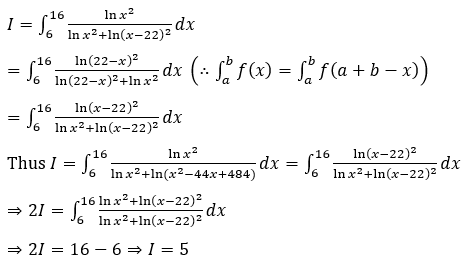Question 7: A wire of length 20 is cut into two parts one is made into a regular hexagon of side a and the other to square. Find ‘a’ if the combined area of a square and regular hexagon is minimum.

Answer: a = 10/(2√3 + 3)

4b + 6a=20

⇒ b=(20-6a)/4 = 5 – (3/2) a

A = b2 + (3√3 a2)/2

A = (5 – (3/2) a) 2 + (3√3 a2)/2

dA/db = 3√3a – 3/2×2(5 – 3a/2) = 0

⇒ 3√3 a + 9/2 a = 15

⇒ 2√3 a + 3a=10

⇒ a = 10/(2√3 + 3)

Question 8: If x2 + y2 + px + y(1 – p) = 0 is the equation of circle, r ∈ (0,5], q = p2 then number of integral values of (p,q) satisfy is

a. 16
b. 14
c. 19
d. 21

Let S : x2 + y2 + px + y(1 – p) = 0

r = √((p/2) 2 + ((1 – p)/2) 2 )

0 < (p2 + (1 – p) 2)/4 ≤ 25

⇒ 0 < 2p2 + 1 – 2p ≤ 100

⇒ 0 < 2p2 – 2p + 1 ≤ 100

⇒ ( – 1/4) < (p – 1/2)2 ≤ 199/4

⇒ (p – 1/2) 2 ≤ (√199/2) 2

Total number of integral pairs of (p,q) = 14

Question 9: In a certain biased die, the probability of getting a particular face is (1/6 + x) and the opposite is (1/6 – x) and 0 < x < 1/6. The probability for other faces is 1/6 each. The sum of opposite faces in a die is 7. If the probability of getting a sum 7 in a throw of 2 such die is 13/96, then x is.

a. 1/8
b. 1/12
c. 1/18
d. 1/20

For particular and opposite faces, if sum is 7.

Probability = 2(1/6 + x)(1/6 – x)

For remaining faces in two die, if sum is 7,

Probability = 2(1/6 x 1/6 + 1/6 x 1/6)

Given, 2[(1/6 + x)(1/6 – x) + 1/6 x 1/6 + 1/6 x 1/6] = 13/96

⇒ 1/36 – x2 + 2/36 = 13/(96×2)

⇒ 1/12 – x2 = 13/(96×2)

⇒ x2 = 1/12 – 13/(96×2) = 1/12 (1 – 13/16) = 1/12 ((16 – 13)/16)

Therefore, x = 1/8

Question 10:Find y(π) if y(0) = 7 and dy/dx = 2(y – 2 sin x – 10)x + 2 cos x

Answer: y(π) = 3eπ^2 + 10

dy/dx = 2(y – 2 sin x – 10)x + 2 cos x

dy/dx – 2 cos x = 2(y – 2 sin x – 10)x

(d/dx (y – 2 sin x – 10))/((y – 2 sin x – 10) ) = 2x

⇒ ∫d(y – 2 sin x – 10)/((y – 2 sin x – 10) ) = ∫2x dx

⇒ log |y – 2 sin x – 10| = x2 + C

At x = 0, y = 7

⇒ log |7 – 0 – 10| = 0 + C⇒ C = log 3

At x = π

⇒ log |y – 2 sin π – 10| = π2 + log 3

⇒ log ((y – 10)/3) = π2

⇒ y(π) = 3eπ^2 + 10

Question 11:If α, β are distinct roots of x^2 + bx + c = 0 then find

$$\begin{array}{l}\lim_{x \rightarrow \beta}\frac{e^{2(x^2+bx+c)} – 1 – 2(x^2 + bx + c)}{(x-\beta)^2}\end{array}$$

Answer: 2(β – α)2 = 2(b2 – 4c)

$$\begin{array}{l}\lim_{x \rightarrow \beta}\frac{e^{2(x^2+bx+c)} – 1 – 2(x^2 + bx + c)}{(x-\beta)^2}\end{array}$$

=

$$\begin{array}{l}\lim_{x \rightarrow \beta}\frac{e^{2(x-\alpha)(x-\beta)} – 1 – 2(x-\alpha)(x-\beta)}{(x-\beta)^2(x-\alpha)^2} \times (x-\alpha)^2\end{array}$$

Let (x – α)(x – β) = y

then

$$\begin{array}{l}\lim_{y \rightarrow 0} \frac{e^{2y}-1-2y}{y^2} \times (\beta – \alpha)^2\end{array}$$

Using L hospital rule,

=

$$\begin{array}{l}(\beta – \alpha)^2 \lim_{y \rightarrow 0} \frac{2e^{2y}-2}{2y}\end{array}$$

=

$$\begin{array}{l}(\beta – \alpha)^2 \lim_{y \rightarrow 0} \frac{e^{2y}-1}{2y} \times 2\end{array}$$

This implies, 2(β – α)2 = 2(b2 – 4c)

Question 12:If (z + i)/(z + 2i) is purely real, then the locus of z is

a. x – axis
b. y – axis
c. y = x
d. y = x/2

(z + i)/(z + 2i) is purely real, so (z + i)/(z + 2i) =

$$\begin{array}{l}(\overline{\frac{z + i}{z + 2i}})\end{array}$$

$$\begin{array}{l}z\bar{z} – 2iz + i\bar{z} + 2 = z\bar{z} – iz + 2i\bar{z} + 2\end{array}$$

$$\begin{array}{l}z(-i) + \bar{z}(-i) = 0\end{array}$$

$$\begin{array}{l}z = -\bar{z}\end{array}$$

z is purely imaginary

Therefore, z lies on y – axis.

Question 13:Tangent and normal at P(2, -4) to parabola y2 = 8x meet directrix at A and B. Q(a,b) is such that APBQ is a square. Find 2a + b.

a.-12
b.-16
c.-20
d.-18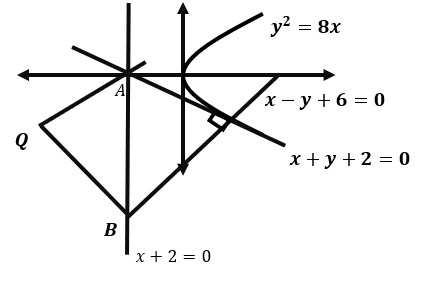y2 = 8x

⇒ a = 2

Tangent equation

-4y = 4(x+2)⇒ x+y+2 = 0

Normal: x-y+6 = 0

Directrix x+2 = 0

A = (-2, 0), B = (-2, -8)

Because, mid-point AB is (-2, -4)

So, Q = (-6, -4)

2a+b = -16

Question 14:If y = log x + log x(1/3) + log x(1/9) + .. and (2 + 4 + 6 + ⋯ + 2y)/(3 + 6 + 9 + ⋯ + 3y) = 1/9 log x then the values of x and y are

a. x = 105, y = 8
b. x = 106, y = 9
c. x = 106, y = 8
d. x = 105, y = 9

y = log x + log x(1/3) + log x(1/9) + ⋯

y = (1 + 1/3 + 1/9 + ⋯) log x

y = [1/(1 – 1/3)] log x = 3/2 log x ⋯(i)

(2 + 4 + 6 + ⋯ + 2y)/(3 + 6 + 9 + ⋯ + 3y) = 2(1 + 2 + 3 + ⋯ + y)/3(1 + 2 + 3 + ⋯ + y)

⇒2/3 = (1/9) log x

log x = 6

x = 106

y = 3/2 log x ⋯ From (i)

y = 3/2×6 = 9

Question 15:In a ∆ABC, if sinA/sinB = sin(A-b)/sin(A-c) and a,b,c are the sides of the ∆ABC, then the correct relationship between a,b,c is ___.

a. c2 (a2 + b2 ) = a2 (c2 – b2)
b. a2 (b2 – c2 ) = b2 (a2 – c2)
c. ac(a2 – c2 ) = b2 (a2 – b2)
d. bc(a2 – c2 ) = a2 (b2 – c2)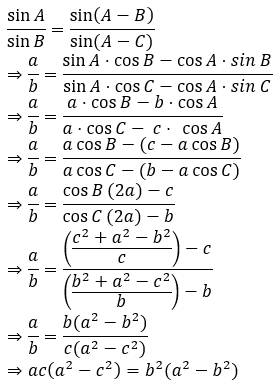Question 16:A plane has equation x – y + z = 5 and a line has direction ratios as (2,3,-6), then the distance of the point P(1,3,5) along the line from the given plane is:

a. 2 m
b. 2√3 m
c. 3m
d. 3√2 m

Equation of line: (x – 1)/2 = (y – 3)/3 = (z – 5)/( – 6) = k

⇒ x = 2k + 1 , y = 3k + 3 , z = – 6k + 5

Equation of plane: x – y + z = 5

⇒ 2k + 1 – 3k – 3 – 6k + 5 = 5

⇒ – 7k = 2 ⇒ k = – 2/7

(x, y, z)≡ (3/7, 15/7, 47/7)

$$\begin{array}{l}d = \sqrt{(4/7)^2 + (6/7)^2 + ( – 12/7)^2 }\end{array}$$

d = 1/7 √(16 + 36 + 144)

d = 14/7 = 2

Question 17:In (sin-1 x )2 – (cos-1 x )2 = a for 0 < x < 1. Then find 2x2 – 1.

a. sin(2a/π)
b. cos(4a/π)
c. cos(2a/π)
d. sin(4a/π)

(sin-1 x )2 – (cos-1 x )2 = a

⇒ (sin-1 x )2 – (π/2 – sin-1 x )2 = a

⇒(sin-1 x )2 – (π2/4) – (sin-1x )2 + π sin-1 x = a

⇒ π sin-1 x = a + π2/4

⇒x = sin (a/π + π/4)

Now, 2x2 – 1 = 2 sin2(a/π + π/4) – 1

= – cos(2a/π + π/2)

= sin(2a/π)

Question 18:If (-2, 2) satisfies y + x (dy/dx) = x2, then the correct equation is

a. x2 + 2xy + 12 = 0
b. x2 + 2xy – 12 = 0
c. x2 + 2xy + 4 = 0
d. x3 – 3xy – 4 = 0

Solution:

x=-2 and y=2

y + x( dy/dx) = x2

⇒ y dx+x dy = x2 dx

⇒∫d(xy) =∫ x2 dx

⇒ x y = x3/3 + c

At x=-2 & y=2

⇒ -4 = (-8)/3 + c

⇒c = -4 + 8/3

Therefore, xy = x3/3 – 4/3

⇒3xy = x3 – 4 ⇒x3 – 4 – 3xy = 0

Question 19: Solve

$$\begin{array}{l}\int \frac{1}{(x^2+x+1)^2}dx\end{array}$$

a.
$$\begin{array}{l}\frac{4}{\sqrt{3}} tan^{-1}(\frac{2x-1}{\sqrt{3}}) + \frac{\sqrt{3}}{4}(\frac{2x-1}{x^2+x+1})+c\end{array}$$

b.
$$\begin{array}{l}\frac{4}{3\sqrt{3}} tan^{-1}(\frac{2x-1}{\sqrt{3}}) – \frac{\sqrt{3}}{4}(\frac{2x-1}{x^2+x+1})+c\end{array}$$

c.
$$\begin{array}{l}\frac{4}{3\sqrt{3}} tan^{-1}(\frac{2x+1}{\sqrt{3}}) + \frac{1}{3}(\frac{2x+1}{x^2+x+1})+c\end{array}$$

d.
$$\begin{array}{l}\frac{4}{3\sqrt{3}} tan^{-1}(\frac{2x+1}{\sqrt{3}}) – \frac{1}{3}(\frac{2x+1}{x^2+x+1})+c\end{array}$$